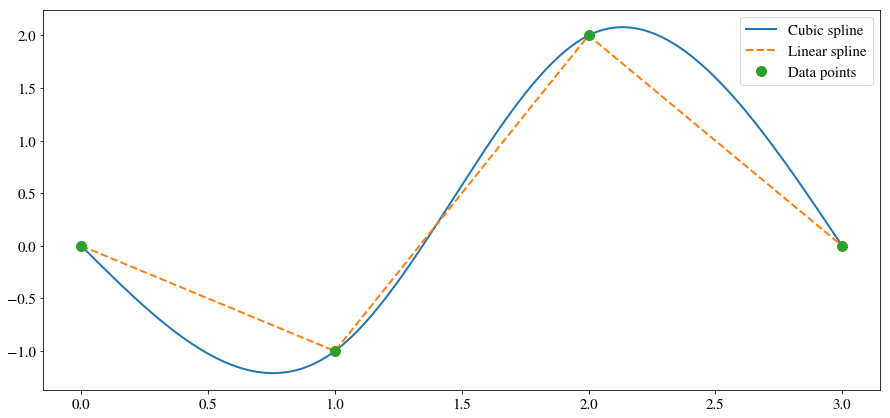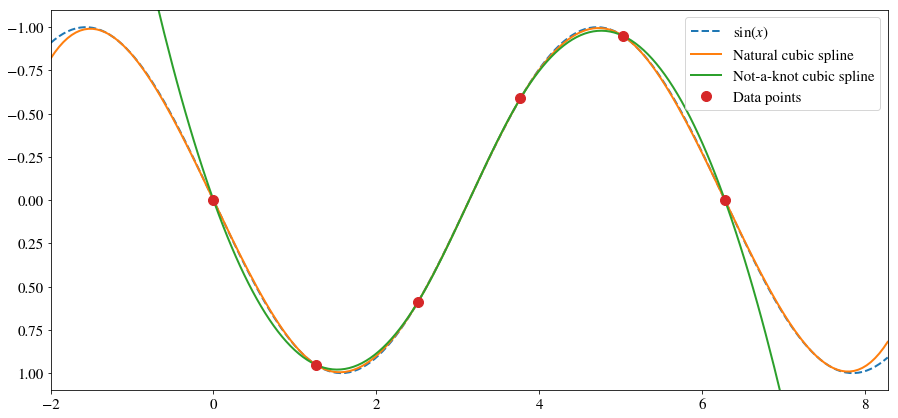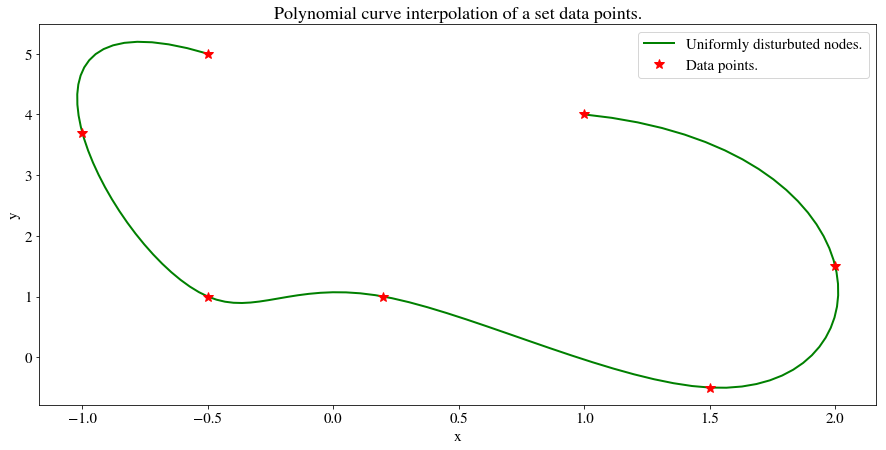# Cubic Splines¶

### Modules - Curve Fitting¶

Last edited: January 26th 2018

Splines is a type of data interpolation, a method of (re)constructing a function between a given set of data points. Interpolation can be used to represent complicated and computationally demanding functions as e.g. polynomials. Then, using a table of a few function evaluations, one can easily approximate the true function with high accuracy.

In spline interpolation the data is interpolated by several low-degree polynomials. This differs from polynomial interpolation, in which the data is interpolated by a single polynomial of a high order. For a general discussion on polynomial interpolation we refer you to our notebook on polynomial interpolation. The simplest example of spline interpolation is linear splines, where the data points are simply connected by straight lines. We are going to discuss interpolation by cubic splines, which interpolates the polynomials using cubic polynomials with continuous first and second derivatives. We will create an algorithm and some functions for computing a cubic spline.

We start by importing needed packages and setting common figure parameters.

In :
import numpy as np
import matplotlib.pyplot as plt
import scipy.sparse as sp
import scipy.linalg as la

%matplotlib inline

# Set some figure parameters
newparams = {'figure.figsize': (15, 7), 'axes.grid': False,
'lines.markersize': 10, 'lines.linewidth': 2,
'font.size': 15, 'mathtext.fontset': 'stix',
'font.family': 'STIXGeneral'}
plt.rcParams.update(newparams)


## A Simple Example¶

Assume that we are given four data points: $\{(0, 0), (1, -1), (2, 2), (3, 0)\}$. The cubic spline interpolating these points is $$S(x) = \begin{cases} -\frac{12}{5}x + \frac{7}{5}x^3, & 0\leq x < 1,\\ -1 + \frac{9}{5}(x - 1) + \frac{21}{5}(x-1)^2 - 3(x-1)^3, & 1 \leq x < 2,\\ 2 + \frac{6}{5}(x - 2) -\frac{24}{5}(x-2)^2 + \frac{8}{5}(x-2)^3, & 2 \leq x < 3.\\ \end{cases}$$ Let's plot the data points, linear spline and cubic spline!

In :
n = 200
x1 = np.linspace(0, 1, n)
x2 = np.linspace(1, 2, n)
x3 = np.linspace(2, 3, n)

# Cubic spline
S1 = -12/5*x1 + 7/5*x1**3
S2 = -1 + 9/5*(x2 - 1) + 21/5*(x2 - 1)**2 - 3*(x2 - 1)**3
S3 = 2 + 6/5*(x3 - 2) - 24/5*(x3 - 2)**2 + 8/5*(x3 - 2)**3
plt.plot(np.concatenate([x1, x2, x3]),  np.concatenate([S1, S2, S3]), label="Cubic spline")
# Linear spline
plt.plot([0, 1, 2, 3], [0, -1, 2, 0], "--", label="Linear spline")
# Data points
plt.plot([0, 1, 2, 3], [0, -1, 2, 0], "o", label="Data points")

plt.legend()
plt.show()Exercise: Check that the cubic spline above has continuous first and second derivatives.

## Definition¶

A general cubic spline $S(x)$ interpolating the $n$ data points $\{(x_1,y_1), (x_2, y_2),..., (x_n, y_n)\}$ can be written as

\begin{equation} S(x) = \begin{cases} S_1(x) &= y_1 + b_1(x - x_1) + c_1(x-x_1)^2 + d_1(x-x_1)^3, & \text{for } x\in[x_1, x_2],\\ S_2(x) &= y_2 + b_2(x - x_2) + c_2(x-x_2)^2 + d_2(x-x_2)^3, & \text{for } x\in[x_2, x_3],\\ &\vdots&\\ S_{n-1} (x) &= y_{n-1} + b_{n-1}(x - x_{n-1}) + c_{n-1}(x-x_{n-1})^2 + d_{n-1}(x-x_{n-1})^3, & \text{for } x\in[x_{n-1}, x_n],\\ \end{cases} \label{eq:spline} \end{equation}

for some constants $b_i, c_i, d_i$, $i=1, ..., n$. As mentioned in the introduction, we demand that the spline in continuous and has continuous first and second derivatives. This gives the following properties: 

1. $S_i(x_i)=y_i$ and $S_i(x_{i+1})=y_{i+1}$ for $i=1,...,n-1$,
2. $S_{i-1}'(x_i)=S_{i}'(x_i)$ and $S_i(x_{i+1})=y_{i+1}$ for $i=2,...,n-1$,
3. $S_{i-1}''(x_i)=S_{i}''(x_i)$ and $S_i(x_{i+1})=y_{i+1}$ for $i=2,...,n-1$.

The three properties make sure that the spline is continuous and smooth.

The total number of constants $b_i, c_i, d_i$ that we need to compute is $3(n-1)$.

## Endpoint conditions¶

Note that the total number of conditions imposed by the properties above is $3n-5$. However, the total number of coefficients $b_i, c_i, d_i$ we need to compute is $3(n-1)$. Hence, we need two additional conditions to make the spline $S(x)$ unique. This is achieved thru endpoint conditions.

There are several choices of endpoint conditions (see e.g. ). We will be considering natural cubic splines,

4a. $S''_1(x_1)= 0$ and $S''_{n-1}(x_n)=0$,

and not-a-knot cubic splines

4b. $S_1'''(x_2)=S_2'''(x_2), \; S_{n-2}'''(x_{n-1})=S_{n-1}'''(x_{n-1})$.

Exercise: Which endpoint condition is used in the example above?

## Algorithm¶

From property 1, 2 and 3 we obtain

\begin{equation} y_{i+1} = y_{i}+b_{i}(x_{i+1}-x_i) + c_i(x_{i+1}-x_i)^2 + d_i(x_{i+1}-x_i)^3, \quad i=1,...,n-1, \label{eq:prop1} \end{equation}\begin{equation} 0 = b_i + 2c_i(x_{i+1}-x_i) + 3d_i(x_{i+1}-x_i)^2-b_{i+1}, \quad i=1,...,n-2, \label{eq:prop2} \end{equation}

and

\begin{equation} 0 = c_i+3d_i(x_{i+1}-x_i)-c_{i+1}, \quad i=1,...,n-2, \label{eq:prop3} \end{equation}

respectively. The derivation is straight forward, and is left as an exercise for the reader. If we solve these equations, we obtain the constants $b_i, c_i, d_i$ and thus the cubic spline. To simplify the notation, we define $\Delta x_i=x_{i+1}-x_i$ and $\Delta y_i = y_{i+1}-y_i$. By using equation \eqref{eq:prop1} and \eqref{eq:prop3} we obtain the following expressions for $b_i$ and $d_i$ in terms of the $c$-coefficients:

\begin{align} d_i &= \frac{c_{i+1}-c_i}{3\Delta x_i}, \label{eq:d}\\ b_i &= \frac{\Delta y_i}{\Delta x_i}-\frac{1}{3}\Delta x_i (2 c_i + c_{i+1}).\label{eq:b} \end{align}

If we insert this into equation \eqref{eq:prop2} we obtain,

$$\Delta x_ic_i + 2(\Delta x_i + \Delta x_{i+1})c_{i+1}+\Delta x_{i+2}c_{i+2} = 3\left(\frac{\Delta y_{i+1}}{\Delta x_{i+1}}-\frac{\Delta y_{i}}{\Delta x_{i}}\right)$$

which is $n-2$ equations for $c_1,..., c_n$. The natural spline endpoint condition gives $c_1=c_n=0$. We can write this as the matrix equation

$$\begin{pmatrix} 1 & 0 & 0 & 0 & \cdots & 0 & 0 \\ \Delta x_1 & 2\Delta x_1 + 2\Delta x_2 & \Delta x_2 & 0 & \cdots&0&0\\ 0 & \Delta x_2 & 2\Delta x_2 + 2\Delta x_3 & \Delta x_3 & \cdots&0&0\\ \vdots & \vdots & \ddots & \ddots & \ddots & \vdots & \vdots\\ 0 & 0 & 0 & 0 & \Delta x_{n-2} & 2\Delta x_{n-2} + 2\Delta x_{n-1} & \Delta x_{n-1}\\ 0 & 0 & 0 & 0 & 0 & 0 & 1 \end{pmatrix} \begin{pmatrix} c_1 \\ c_2 \\ \\ \vdots \\ \\ c_n \end{pmatrix} = \begin{pmatrix} 0 \\ 3\left(\frac{\Delta y_{2}}{\Delta x_2}-\frac{\Delta y_1}{\Delta x_1}\right) \\ \vdots \\ 3\left(\frac{\Delta y_{n-1}}{\Delta x_{n-1}}-\frac{\Delta y_{n-2}}{\Delta x_{n-2}}\right) \\ 0 \end{pmatrix}.$$

The algorithm for finding the spline is now quite apparent. We start by constructing the matrix equation, then solve it to find $c_i$ and in turn compute $b_i$ and $d_i$ via the equations \eqref{eq:d} and \eqref{eq:b}.

The first and last row of the matrix is altered with the not-a-knot endpoint conditions. Note that property 4b implies $d_1=d_2$ and $d_{n-2}=d_{n-1}$. If we insert this into equation \eqref{eq:d} for $d_i$, we obtain $$\Delta x_2 c_1 -(\Delta x_1 + \Delta x_2) c_2 + \Delta x_1 c_3 = 0,$$ $$\Delta x_{n-1} c_{n-1} -(\Delta x_{n-2} + \Delta x_{n-1})c_{n-1}+\Delta x_{n-2}c_n = 0.$$ The first row in with the not-a-knot end conditions becomes $$(\Delta x_2\;\; -(\Delta x_1 + \Delta x_2)\;\; \Delta x_1\;\; 0\;\; 0\;\; ...).$$ Likewise, the last row becomes $$(0\;\; ... \;\; 0 \;\; \Delta x_{n-1}\;\; -(\Delta x_{n-2} + \Delta x_{n-2})\;\; \Delta x_{n-2}).$$

We now proceed to create a function that computes the cubic spline that interpolates the points $\{(x_1,y_1), (x_2, y_2),..., (x_n, y_n)\}$. Note that the matrix equation above is tridiagonal, and it can therefore be stored as a $3\times n$ array and solved effectively by using scipy.linalg.solve_banded. In the not-a-knot-case the matrix becomes a banded matrix with two upper and lower diagonals.

Exercise: Derive equations \eqref{eq:prop1}, \eqref{eq:prop2} and \eqref{eq:prop3} from the properties 1-3.

In :
def cubic_spline_coeffs(x, y, endpoint="natural"):
""" Computes the coefficients in the cubic spline that interpolates
the points (x_1,y_1), (x_2, y_2),..., (x_n, y_n).
Parameters:
x: array_like, shape (n>2,).
x-value of the points being interpolated. Values must be
real and in strictly increasing order.
y: array_like, shape (n>2,)
y-value of the points being interpolated. Values must be
real.
Returns:
array, shape (3, n). The coefficients b, c and d, stored in
the first, second and third row, respectively.
"""
x = np.asarray(x)
y = np.asarray(y)
n = len(x)
dx = np.diff(x)
dy = np.diff(y)

# Find the vector for the right hand side
rhs = np.zeros(n)
rhs[1:-1] = 3*(dy[1:]/dx[1:] - dy[:-1]/dx[:-1])

# Compute the matrix and store a matrix diagonal ordered form
if (endpoint == "natural"):
matrix = np.zeros((3, n))
bands = (1, 1)
matrix[1, 1:-1] = 2*(dx[:-1] + dx[1:]) # Diagonal
matrix[1, 0] = matrix[1, -1] = 1
matrix[0, 2:] = dx[1:] # Upper diagonal
matrix[2, :-2] = dx[:-1] # Lower diagonal

if (endpoint == "not-a-knot"):
matrix = np.zeros((5, n))
bands = (2, 2)
matrix[2, 1:-1] = 2*(dx[:-1] + dx[1:]) # Diagonal
matrix[1, 2:] = dx[1:] # Upper diagonal
matrix[3, :-2] = dx[:-1] # Lower diagonal
# First row
matrix[2, 0] = dx
matrix[1, 1] = -dx - dx
matrix[0, 2] = dx
# Last row
matrix[2, -1] = dx[-2]
matrix[3, -2] = -dx[-2] - dx[-3]
matrix[4, -3] = dx[-1]

# Call a solver for a banded matrix
c = la.solve_banded(bands, matrix, rhs,
overwrite_ab=True, overwrite_b=True, check_finite=False)

# Find the remainding coefficients
d = np.diff(c)/(3*dx)
b = dy/dx - dx*(2*c[:-1] + c[1:])/3

return b, c, d


We also need a function that can evaluate the spline given the coefficients.

In :
def cubic_spline_eval(x, xdata, ydata, b, c, d):
""" Evaluates the cubic spline that interpolates {(xdata, ydata)}
at x with coefficients b, c and d.
Parameters:
x:          array_like, shape(m,).
x-values (axis) at which the spline is evaluated.
a, b, c, d: array_like, shapes (n,), (n-1,), (n,) and (n-1,).
Coefficients of the spline.
Return:
array, shape(m,). Function evaluation of the spline.
"""
x = np.asarray(x)
y = np.zeros(len(x))
m = 0
for i in range(len(xdata) - 1):
n = np.sum(x < xdata[i + 1]) - m
xx = x[m:m + n] - xdata[i]
y[m:m + n] = ydata[i] + b[i]*xx + c[i]*xx**2 + d[i]*xx**3
m = m + n
xx = x[m:] - xdata[-2]
y[m:] = ydata[-2] + b[-1]*xx + c[-2]*xx**2 + d[-1]*xx**3
return y


## Example¶

We are now ready to find the cubic spline of a general set of data points. To get some basis for comparison, we will interpolate some points from $\sin(x)$.

In :
def func(x):
return np.sin(x)

xdata = np.asarray([0, 2, 4, 6, 8, 10])*np.pi/5
ydata = func(xdata)

x = np.linspace(xdata - 2, xdata[-1] + 2, 200)
y = func(x)

b, c, d = cubic_spline_coeffs(xdata, ydata, "natural")
ya = cubic_spline_eval(x, xdata, ydata, b, c, d)

b, c, d = cubic_spline_coeffs(xdata, ydata, "not-a-knot")
yb = cubic_spline_eval(x, xdata, ydata, b, c, d)

plt.figure()
plt.plot(x, y, "--", label=r"$\sin(x)$")
plt.plot(x, ya, label="Natural cubic spline")
plt.plot(x, yb, label="Not-a-knot cubic spline")
plt.plot(xdata, ydata, 'o', label="Data points")
plt.xlim(x, x[-1])
plt.ylim(np.max(y)*1.1, np.min(y)*1.1)
plt.legend()
plt.show()Note that we have defined the cubic splines outside the domain of the data points. In the not-a-knot cubic spline outer polynomials are extended. That is, the polynomial at $x<x_1$ is the same as for $x_1<x<x_2$. The natural cubic spline on the other hand, defines a polynomial with "opposite curvature" outside the data points. That is, if the spline curves away from the axis at $x_1<x<x_2$ it will curve towards the axis at $x<x_1$.

## Interpolating parameterized curves¶

It is also possible to interpolate parameterized curves with splines. This is done by performing the interpolation on both the x-axis and y-axis as functions of the curve parameter. Consider the following example:

In :
# Define some data points
xdata = [-0.5,-1.0,-0.5, 0.2, 1.5, 2.0, 1.0]
ydata = [ 5.0, 3.7, 1.0, 1.0,-0.5, 1.5, 4.0]
n = len(xdata)

# Parameter values
t = np.linspace(0,1,100)

# Curve interpolation using uniformly disturbuted parameter nodes
ti = np.linspace(0,1,n)
# x-axis
b, c, d = cubic_spline_coeffs(ti, xdata, "not-a-knot")
Px = cubic_spline_eval(t, ti, xdata, b, c, d)
# y-axis
b, c, d = cubic_spline_coeffs(ti, ydata, "not-a-knot")
Py = cubic_spline_eval(t, ti, ydata, b, c, d)

plt.figure
plt.plot(Px,Py,'g',label='Uniformly disturbuted nodes.')
plt.plot(xdata,ydata,'r*',label='Data points.')
plt.title('Polynomial curve interpolation of a set data points.')
plt.legend(), plt.xlabel('x'), plt.ylabel('y')
plt.show()Exercise: Use the Chebychev nodes instead of the uniformly distributed parameter nodes. Hint. See the polynomial interpolation notebook.

## Why Cubic Splines?¶

There are several benefits of using cubic splines opposed to e.g. a high order polynomial interpolation or higher order splines. In our notebook on polynomial interpolation we showed that large oscillations (and thus large errors) may occur when approximating a function using a high order polynomial. This is called Runge's phenomenon. We showed that the error due to this oscillation is minimized when using so-called Chebyshev nodes as base for the interpolation. Another workaround is to use low order polynomial splines.

You may be wondering why we don't use a quadratic spline instead of cubic splines. Approximating a function using more function evaluations and higher order polynomials must always be better, right? Wrong! We may of course argue that higher order polynomial splines gives smoother curves, since we are demanding that higher order derivatives are continuous. This may, however, lead to larger errors due to Runge's phenomenon. In addition, higher order polynomial interpolations require more data points. The global change in the curve due to the change in a single data point is thus increased in higher order polynomial splines.